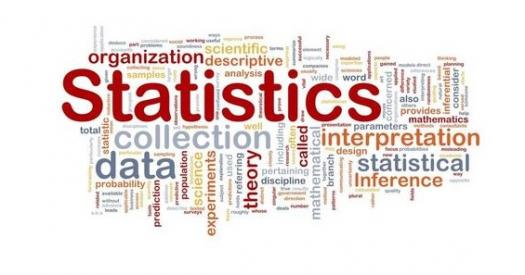# Statistics Internal Examination

10 Questions | Total Attempts: 116SettingsFYBSc Computer ScienceInternal ExaminationStatisticsTotal Marks : 20Max. Time : 20 Min

• 1.
The Variable that does not really have evaluative distinction between its values is measured on______
• 2.
Measurement of Gender is qualitative data
• A.

True

• B.

False

• 3.
Sources of Data
• A.

Primary

• B.

Discrete

• C.

Secondary

• D.

Continuous

• 4.
Collection of data about all the units in the population
• A.

Population Survey

• B.

Census Survey

• C.

Statistical

• D.

Variable

• 5.
Classification of data on the basis of Characteristics
• A.

Attribute

• B.

Chronological

• C.

Variable

• D.

Geograaphical

• 6.
Find the Class Width of Interval 5.25 - 11.25 is ____
• 7.
Smooth Hand Free Curve nearest to all the points is____
• 8.
A data value = Stem Value x Stem Size + Leaf Value x Leaf size
• A.

True

• B.

False

• 9.
Arithmetic Mean is given by
• A.

Addition of all the values and divide by total number of observation

• B.

Addition of all the f.x and divide by total frequency

• C.

Subraction of Values and multiply by total number of observation

• D.

• 10.
In quartiles, central tendency median to be measured must lie in
• A.

First quartile

• B.

Second quartile

• C.

Third quartile

• D.

Four quartile

Related Topics